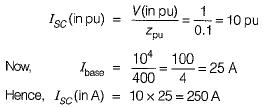Courses

# Fault Analysis Of Power Systems

## 10 Questions MCQ Test Topicwise Question Bank for GATE Electrical Engineering | Fault Analysis Of Power Systems

Description
This mock test of Fault Analysis Of Power Systems for Electrical Engineering (EE) helps you for every Electrical Engineering (EE) entrance exam. This contains 10 Multiple Choice Questions for Electrical Engineering (EE) Fault Analysis Of Power Systems (mcq) to study with solutions a complete question bank. The solved questions answers in this Fault Analysis Of Power Systems quiz give you a good mix of easy questions and tough questions. Electrical Engineering (EE) students definitely take this Fault Analysis Of Power Systems exercise for a better result in the exam. You can find other Fault Analysis Of Power Systems extra questions, long questions & short questions for Electrical Engineering (EE) on EduRev as well by searching above.
QUESTION: 1

### When all the three phases are short circuited it gives rise to

Solution:

A three-phase fault is a symmetrical fault.

QUESTION: 2

### The zero sequence reactance will be more than the positive sequence reactance, if the equipment is:

Solution:

For transmission line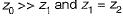QUESTION: 3

### The sequence networks for a three-phase faults are connected in

Solution:

A three-phase fault is a symmetrical fault where only positive sequence network (current exists).

QUESTION: 4

Match List-l (Symbol for 3-phase transformer connections) with List-ll (Zero-sequence equivalent circuits) and select the correct answer using the codes given below the lists:
List-I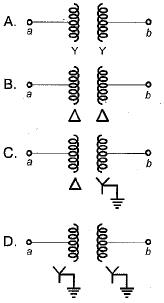List-ll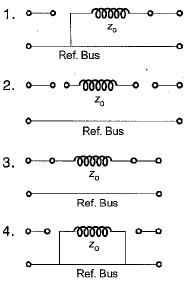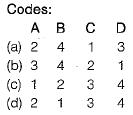Solution:

Using switch diagram, we find the zero sequence network for a transformer.

QUESTION: 5

The base impedance and base voltage for a given power system are 10Ω and 400 V respectively. The base kVA and the base current will be respectively

Solution: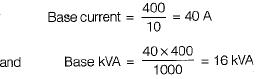QUESTION: 6

The per-unit admittance YPu of a power system in terms of the base voltage Vbase and the base voltamperes (VA)base is given by

Solution: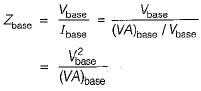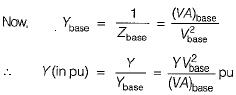QUESTION: 7

In a line to ground fault on a system, the fault current is 1500 A. The zero sequence current is

Solution: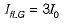or,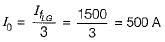QUESTION: 8

The fault current, in a double line to ground fault on a system depends on

Solution: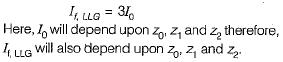QUESTION: 9

There may be a zero sequence components in
1. line voltage of star circuit
2. phase voltage of star circuit
3. line current of delta circuit
4. phase current of delta circuit
Select the correct answer from the given options:

Solution:
QUESTION: 10

A 10 kVA, 400 V/200V single phase transformer with 10% impedance draws a steady short circuit line current of

Solution: